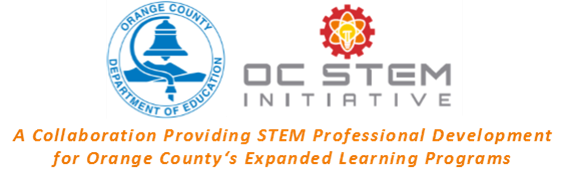## Supervisors - Online

Self-reflection is critical to the success of implementing effective programs. This reflection tool was developed to be used by Expanded Learning Supervisors to help strengthen their staff's ability to deliver their lessons. This tool can be used as often as you'd like; after observing one particular lesson, or after an on-going project has concluded. We hope you are able to strengthen your own skill set, and support your staff better, through this process and think about new ways to provide quality STEM to students.

### Question Title

#### * 4. Think about the last STEM Project/Activity you observed, how were the students reacting to the activity?

 0 Students 1-4 Students 5-10 Students 11-15 Students 16 + Students Number of Students Participating in the Activity Number of Students Participating in the Activity 0 Students Number of Students Participating in the Activity 1-4 Students Number of Students Participating in the Activity 5-10 Students Number of Students Participating in the Activity 11-15 Students Number of Students Participating in the Activity 16 + Students Number of Student Asking Questions Number of Student Asking Questions 0 Students Number of Student Asking Questions 1-4 Students Number of Student Asking Questions 5-10 Students Number of Student Asking Questions 11-15 Students Number of Student Asking Questions 16 + Students Number of Students You Overhead Having Conversations That Directly Related to the Activity Topic Number of Students You Overhead Having Conversations That Directly Related to the Activity Topic 0 Students Number of Students You Overhead Having Conversations That Directly Related to the Activity Topic 1-4 Students Number of Students You Overhead Having Conversations That Directly Related to the Activity Topic 5-10 Students Number of Students You Overhead Having Conversations That Directly Related to the Activity Topic 11-15 Students Number of Students You Overhead Having Conversations That Directly Related to the Activity Topic 16 + Students Number of Students That Completed The Task Number of Students That Completed The Task 0 Students Number of Students That Completed The Task 1-4 Students Number of Students That Completed The Task 5-10 Students Number of Students That Completed The Task 11-15 Students Number of Students That Completed The Task 16 + Students Number of Students That Had Directly Influenced the Decision To Conduct the Activity Number of Students That Had Directly Influenced the Decision To Conduct the Activity 0 Students Number of Students That Had Directly Influenced the Decision To Conduct the Activity 1-4 Students Number of Students That Had Directly Influenced the Decision To Conduct the Activity 5-10 Students Number of Students That Had Directly Influenced the Decision To Conduct the Activity 11-15 Students Number of Students That Had Directly Influenced the Decision To Conduct the Activity 16 + Students Number of Students That Recognize the STEM Learning Goal (Outcome) Number of Students That Recognize the STEM Learning Goal (Outcome) 0 Students Number of Students That Recognize the STEM Learning Goal (Outcome) 1-4 Students Number of Students That Recognize the STEM Learning Goal (Outcome) 5-10 Students Number of Students That Recognize the STEM Learning Goal (Outcome) 11-15 Students Number of Students That Recognize the STEM Learning Goal (Outcome) 16 + Students Number of Students That Can Articulate the STEM Learning Goal (Outcome) Number of Students That Can Articulate the STEM Learning Goal (Outcome) 0 Students Number of Students That Can Articulate the STEM Learning Goal (Outcome) 1-4 Students Number of Students That Can Articulate the STEM Learning Goal (Outcome) 5-10 Students Number of Students That Can Articulate the STEM Learning Goal (Outcome) 11-15 Students Number of Students That Can Articulate the STEM Learning Goal (Outcome) 16 + Students

T